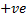# The point (4, 1) undergoes the following three transformation successively i. Reflection about the lineii. Translation through a distance 2 units along the positive direction of-axis iii. Rotation through an angleabout the origin in the counterclockwise direction Then the final position of the point is given by the coordinates a)b)c)d)## Question ID - 52957 :- The point (4, 1) undergoes the following three transformation successively i. Reflection about the lineii. Translation through a distance 2 units along the positive direction of-axis iii. Rotation through an angleabout the origin in the counterclockwise direction Then the final position of the point is given by the coordinates a)b)c)d)3537

(c)

Reflection about the line, changes the point (4, 1) to (1, 4). On translation of (1,4) throughdistance of 2 units alongdirection of-axis the point becomes (1+2, 4), i.e., (3, 4)On rotation about origin through an angle, the pointtakes the positionsuch that. Alsoand. Now,Next Question :
 Linehas interceptsandon the coordinates axes. When the axes are rotated through a given angle keeping the origin fixed, the same linehas interceptsand. Then, a)b)c)d)